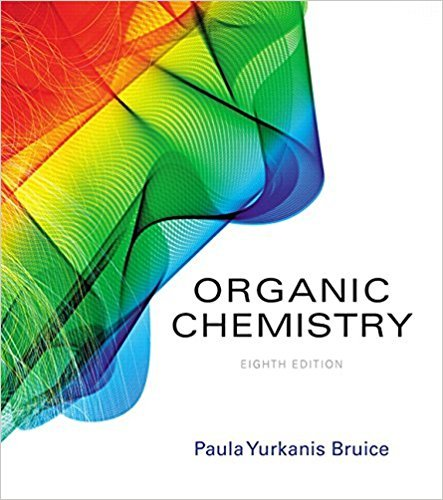×
×

# Solved: A solution prepared by mixing 10 mL of a 0.10 M solution of the R enantiomer ofISBN: 9780134042282 482

## Solution for problem 37 Chapter 4.11

Organic Chemistry | 8th Edition

• Textbook Solutions
• 2901 Step-by-step solutions solved by professors and subject experts
• Get 24/7 help from StudySoup virtual teaching assistantsOrganic Chemistry | 8th Edition

4 5 1 427 Reviews
25
4
Problem 37

A solution prepared by mixing 10 mL of a 0.10 M solution of the R enantiomer of a compound and 30 mLof a 0.10 M solution of the S enantiomer was found to have an observed specific rotation of +4.8. What isthe specific rotation of each of the enantiomers? (Hint: mL * M = millimole, abbreviated as mmol)

Step-by-Step Solution:
Step 1 of 3

Ch. 3 Quantum Theory and Electronic Structures 1. Forms of energy a. Kinetic energy – energy from motion i. Ek = 1/2m(v^2) ii. Kinetic energy = one half of the mass times velocity squared iii. Thermal energy (form of kinetic energy)– associated with the random motion of atoms and molecules b. Potential...

Step 2 of 3

Step 3 of 3

##### ISBN: 9780134042282

The full step-by-step solution to problem: 37 from chapter: 4.11 was answered by , our top Chemistry solution expert on 03/16/18, 04:59PM. Since the solution to 37 from 4.11 chapter was answered, more than 382 students have viewed the full step-by-step answer. The answer to “A solution prepared by mixing 10 mL of a 0.10 M solution of the R enantiomer of a compound and 30 mLof a 0.10 M solution of the S enantiomer was found to have an observed specific rotation of +4.8. What isthe specific rotation of each of the enantiomers? (Hint: mL * M = millimole, abbreviated as mmol)” is broken down into a number of easy to follow steps, and 58 words. This full solution covers the following key subjects: . This expansive textbook survival guide covers 127 chapters, and 2116 solutions. Organic Chemistry was written by and is associated to the ISBN: 9780134042282. This textbook survival guide was created for the textbook: Organic Chemistry, edition: 8.

Unlock Textbook Solution